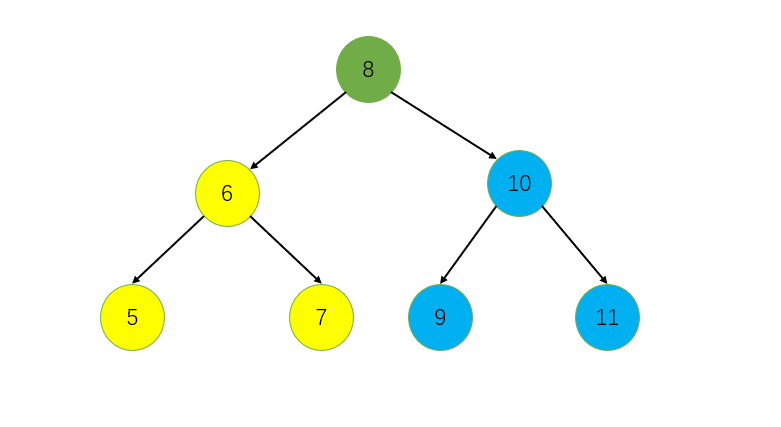PAT 1043 Is It a Binary Search Tree (25分) 由前序遍历得到二叉搜索树的后序遍历

### 题目

A Binary Search Tree (BST) is recursively defined as a binary tree which has the following properties:

• The left subtree of a node contains only nodes with keys less than the node's key.
• The right subtree of a node contains only nodes with keys greater than or equal to the node's key.
• Both the left and right subtrees must also be binary search trees.

If we swap the left and right subtrees of every node, then the resulting tree is called the Mirror Image of a BST.

Now given a sequence of integer keys, you are supposed to tell if it is the preorder traversal sequence of a BST or the mirror image of a BST.

Input Specification:
Each input file contains one test case. For each case, the first line contains a positive integer N (≤1000). Then N integer keys are given in the next line. All the numbers in a line are separated by a space.

Output Specification:
For each test case, first print in a line YES if the sequence is the preorder traversal sequence of a BST or the mirror image of a BST, or NO if not. Then if the answer is YES, print in the next line the postorder traversal sequence of that tree. All the numbers in a line must be separated by a space, and there must be no extra space at the end of the line.

Sample Input 1:
7
8 6 5 7 10 8 11
Sample Output 1:
YES
5 7 6 8 11 10 8
Sample Input 2:
7
8 10 11 8 6 7 5
Sample Output 2:
YES
11 8 10 7 5 6 8
Sample Input 3:
7
8 6 8 5 10 9 11
Sample Output 3:
NO

### 题目解读

• 左子树所有节点的键值小于根节点
• 右子树所有节点的键值大于等于根有点
• 左子树和右子树也必须是二叉搜索树（满足上面两点）

• 左子树所有节点的键值大于等于根节点
• 右子树所有节点的键值小于根有点
• 左子树和右子树也必须满足上面两点

### 思路分析• 当前树根是8，设置左指针i，初始是8的下一个位置，右指针j，初始是当前树最后一个有效位置
• i从左往右，扫描前序序列，遇到比根小的就i++，会停在9的位置；
• j从右往左，扫描前序序列，遇到比根大于或等于的就j--，最后会停在7的位置
• 这样一次扫描后，应该满足 j + 1 == i，对于每一个二叉搜索树的前序遍历都应该满足这个特点，所以这里可以作为函数的一个出口，不满足，就退出
• 划分成左右两部分后，因为我们要得到后序遍历（左，右，中），所以对做左子树进行这个处理，对右子树进行这个处理，把当前树根加入vector，注意顺序不能乱！

• 这个函数需要两个参数，一个是当前树的根，一个是当前树最后一个节点在前序序列中的位置
• 每次双指针扫描结束后，不满足 i == j + 1 就退出
• 最小的子树是只有一个节点，此时这两个参数应该相等，所以函数刚进来的时候，判断一个，如果根的位置 > 最后一个有效位置，就直接退出。

• 把输入序列当作二叉搜索树前序遍历，执行这个函数，得到后序结果，
• 如果所得结果中节点个数与输入一致，直接输出
• 如果不一致，把输入序列当作二叉搜索树镜像树的前序遍历，也就是改变标志位，再执行一次这个函数，得到后序结果
• 再判断所得结果中节点个数是否与输入一致，一致则输出，不一致则输出 NO。

### 完整代码

#include<iostream>
#include<vector>
using namespace std;
// 前序遍历序列，后序遍历序列
vector<int> pre, post;
// 是否当作二叉搜索树的镜像树处理
bool ismirror = false;

// 当前处理的树的根节点在前序序列中的下标
// 当前处理的树最后一个节点在前序序列中的有效位置
void GetPost(int root, int tail) {
// 最小的树就一个节点，root=tail
if (root > tail) return;
int i = root + 1, j = tail;
// 当作二叉搜索树树处理
if (!ismirror) {
// 左孩子都小于根
while (i <= tail && pre[i] < pre[root]) i++;
// 右孩子都大于等于根
while (j > root && pre[root] <= pre[j]) j--;
// 当作二叉搜索树的镜像树处理
} else {
// 左孩子都大于等于根
while (i <= tail && pre[i] >= pre[root]) i++;
// 右孩子都小于根
while (j > root && pre[root] > pre[j]) j--;
}
// 不满足二叉搜索树前序遍历结果特点
if (i != j + 1) return;
// 对左子树执行此操作
GetPost(root + 1, j);
// 对右子树执行此操作
GetPost(i, tail);
// 保存当前根
post.push_back(pre[root]);
}

int main() {
int n;
cin >> n;
pre.resize(n);
for (int i = 0; i < n; ++i) cin >> pre[i];
// 当作前序遍历结果，尝试得到后序结果
GetPost(0, n - 1);
// 所得结果节点个数错误
if (post.size() != n) {
// 改变标志位
ismirror = true;
post.clear();
// 当作镜像树前序遍历结果，尝试获取后序结果
GetPost(0, n - 1);
}
// 所得结果正确
if (post.size() == n) {
cout << "YES" << endl;
cout << post;
for (int i = 1; i < n; ++i) cout << " " << post[i];
// 所得结果依然错误
} else {
cout << "NO";
}
return 0;
}